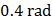# Two coherent sourcesandare separated by a distance four times the wavelengthof the source. The sources lies alongaxis whereas a detector moves alongaxis. Leaving the origin and far off points the number of points where maxima are observed is

## Question ID - 150099 :- Two coherent sourcesandare separated by a distance four times the wavelengthof the source. The sources lies alongaxis whereas a detector moves alongaxis. Leaving the origin and far off points the number of points where maxima are observed is

3537

From,Hereis the path differencefor maximum intensityOrThenNumber of points for maxima becomes 3

Next Question :
 Specific rotation of sugar solution is 0.01 SI units.of impure sugar solution is taken in a polarimeter tube of lengthand an optical rotation ofis observed. The percentage of purity of sugar is the sample is a) 80% b) 89% c) 11% d) 20%